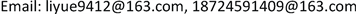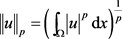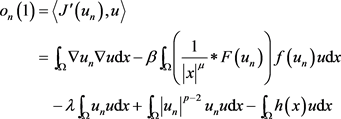﻿ 整数阶Choquard方程三解的存在性 Existence of Three Solutions for a Choquard Equation

Pure Mathematics
Vol. 09  No. 03 ( 2019 ), Article ID: 30129 , 8 pages
10.12677/PM.2019.93039

Existence of Three Solutions for a Choquard Equation

Yue Li, Anran Hou

School of Mathematics, Yunnan Normal University, Kunming YunnanReceived: Apr. 15th, 2019; accepted: Apr. 26th, 2019; published: May 9th, 2019ABSTRACT

We study the following Choquard equation by the Theorem 1.1 in 

$\left\{\begin{array}{l}-\Delta u=\beta \left(\frac{1}{{|x|}^{\mu }}\ast F\left(u\right)\right)f\left(u\right)+\lambda u-{|u|}^{p-2}u+h\left(x\right),\text{}x\in \Omega \\ u=0,\text{}\text{\hspace{0.17em}}\text{}x\in \partial \Omega \end{array}$

where, $\Omega \subset {R}^{3}$ is an open, and bounded domain with a smooth boundary, $h\in {L}^{2}\left(\Omega \right)$ , $0<\mu <3$ , $4 , $\beta >0$ , $\lambda >0$ . Under suitable assumption $f\in C\left(ℝ,ℝ\right)$ , we prove this problem at least three weak solutions.

Keywords:Choquard Equation, Three Critical Points$\left\{\begin{array}{l}-\Delta u=\beta \left(\frac{1}{{|x|}^{\mu }}\ast F\left(u\right)\right)f\left(u\right)+\lambda u-{|u|}^{p-2}u+h\left(x\right),\text{}x\in \Omega \\ u=0,\text{}\text{\hspace{0.17em}}\text{}x\in \partial \Omega \end{array}$1. 引言

$\begin{array}{l}-{\epsilon }^{2}\Delta u+V\left(x\right)u\\ ={\epsilon }^{\mu -N}\left(\frac{1}{{|x|}^{\mu }}\ast F\left(u\right)\right)f\left(u\right)+h\left(u\right),\text{}x\in {ℝ}^{N}\end{array}$ (1.1)(1.2)

$N=3$$q=2$$\mu =1$ 时的情况，是1954年Pekar在  中用来描述极化子静止时的量子理论时提出的。(1.2)式是1976年Choquard在  中描述单组分等离子体的Hartree-Fock理论时提出的。Lions在  中由临界点定理得到方程在 ${H}^{1}\left({ℝ}^{N}\right)$ 中有无穷多镜像解的存在性。对于基态解的一些性质，L. Ma和L. Zhao在  中证明了对于 $q\ge 2$ 时，广义的Choquard方程(1.2)式的每个正解都是径向对称的，并且单调递减到某一点。后来Moroz和Schaftingen在   中消除了这种限制，并得出最佳参数的、基态的正则性和径向对称性，并推导出这些解在无限远处渐近衰减。还有一些人专注于半经典问题，即(1.1)式中的 $\epsilon \to 0$ 。非局部问题(1.1)的半经典解的存在性已经在  中给出。

$\left\{\begin{array}{l}-\Delta u=\beta \left(\frac{1}{{|x|}^{\mu }}\ast F\left(u\right)\right)f\left(u\right)+\lambda u-{|u|}^{p-2}u+h\left(x\right),\text{}x\in \Omega \\ u=0,\text{}x\in \partial \Omega \end{array}$ (1.3)

(f1) $\underset{t\to 0}{\mathrm{lim}}\frac{f\left(t\right)}{t}=0$ .

(f2) $\exists q\in \left(\frac{6-\mu }{3},\mathrm{min}\left\{6-\mu ,\frac{p}{2}\right\}\right)\text{}s.t.\text{}\underset{t\to \infty }{\mathrm{lim}}\frac{f\left(t\right)}{{t}^{q-1}}=0$ .

2. 泛函设置

$\Omega \in {ℝ}^{3}$ 是具有光滑边界的有界开集，Sobolev空间 ${W}_{0}^{1,2}\left(\Omega \right)$ 的范数为

${‖u‖}_{1,2}={\left({\int }_{\Omega }{|\nabla u|}^{2}\text{d}x\right)}^{\frac{1}{2}}$

Lebesgue空间 ${L}^{p}\left(\Omega \right)\text{\hspace{0.17em}}\left(p\ge 1\right)$ 的范数为${\iint }_{{ℝ}^{2N}}\frac{f\left(x\right)h\left(y\right)}{{|x-y|}^{\mu }}\text{d}x\text{d}y\le C\left(r,N,\mu ,t\right){‖f‖}_{r}{‖h‖}_{t}$

(R) $\exists \text{}R>0\text{}s.t.\text{}\underset{u\in \partial {B}_{Y}\left(R\right)}{\mathrm{max}}J\left(u\right)<\underset{u\in Z}{\mathrm{inf}}J\left(u\right)$

(PS) 对任意的序列 $\left\{{u}_{n}\right\}\subset X$ 使得 $\left\{J\left({u}_{n}\right)\right\}\subset ℝ$ 有界，并且 ${‖{J}^{\prime }\left({u}_{n}\right)‖}_{{X}^{\ast }}\to 0$ 有收敛子列。

$J\left(u\right)=\frac{1}{2}{\int }_{\Omega }{|\nabla u|}^{2}\text{d}x-\frac{\beta }{2}{\int }_{\Omega }\left(\frac{1}{{|x|}^{\mu }}\ast F\left(u\right)\right)F\left(u\right)\text{d}x-\frac{\lambda }{2}{\int }_{\Omega }{|u|}^{2}\text{d}x+\frac{1}{p}{\int }_{\Omega }{|u|}^{p}\text{d}x-\frac{1}{2}{\int }_{\Omega }{|h\left(x\right)|}^{2}\text{d}x$

a) $J\in {C}^{1}\left({W}_{0}^{1,2}\left(\Omega \right),ℝ\right)$ 并且满足

$〈{J}^{\prime }\left(u\right),\phi 〉={\int }_{\Omega }\nabla u\nabla \phi \text{d}x-\beta {\int }_{\Omega }\left(\frac{1}{{|x|}^{\mu }}\ast F\left(u\right)\right)f\left(u\right)\phi \text{d}x-\lambda {\int }_{\Omega }u\phi \text{d}x+{\int }_{\Omega }{|u|}^{p-2}u\phi \text{d}x-{\int }_{\Omega }h\left(x\right)\phi \text{d}x$

b) $u\in {W}_{0}^{1,2}\left(\Omega \right)$ 是(1.3)的弱解，当且仅当 $u\in {W}_{0}^{1,2}\left(\Omega \right)$ 是J的临界点。

$f\left(t\right)\le \xi |t|+{C}_{\xi }{|t|}^{q-1},\text{}F\left(t\right)\le \xi {|t|}^{2}+{C}_{\xi }{|t|}^{q}$ (2.1)

$\begin{array}{c}|{\int }_{\Omega }\left(\frac{1}{{|x|}^{\mu }}\ast F\left(u\right)\right)F\left(u\right)\text{d}x|\le C{‖F\left(u\right)‖}_{t}{‖F\left(u\right)‖}_{t}\\ \le C{\left({\int }_{\Omega }{\left({|u|}^{2}+{|u|}^{q}\right)}^{t}\text{d}x\right)}^{\frac{2}{t}}\\ \le C\left({‖u‖}_{2t}^{4}+{‖u‖}_{qt}^{2q}\right)\end{array}$ (2.2)

$\begin{array}{c}J\left(u\right)=\frac{1}{2}{\int }_{\Omega }{|\nabla u|}^{2}\text{d}x-\frac{\beta }{2}{\int }_{\Omega }\left(\frac{1}{{|x|}^{\mu }}\ast F\left(u\right)\right)F\left(u\right)\text{d}x-\frac{\lambda }{2}{\int }_{\Omega }{|u|}^{2}\text{d}x\\ \text{\hspace{0.17em}}\text{\hspace{0.17em}}+\frac{1}{p}{\int }_{\Omega }{|u|}^{p}\text{d}x-\frac{1}{2}{\int }_{\Omega }{|h\left(x\right)|}^{2}\text{d}x\\ \ge \frac{1}{2}{‖u‖}_{1,2}^{2}-C\left({‖u‖}_{2t}^{4}+{‖u‖}_{qt}^{2q}\right)-\frac{\lambda }{2}{‖u‖}_{2}^{2}+\frac{1}{p}{‖u‖}_{p}^{p}-\frac{1}{2}{‖h‖}_{2}^{2}\\ \ge \frac{1}{2}{‖u‖}_{1,2}^{2}-C\left({‖u‖}_{p}^{4}+{‖u‖}_{p}^{2q}+\frac{\lambda }{2}{‖u‖}_{p}^{2}\right)+\frac{1}{p}{‖u‖}_{p}^{p}-\frac{1}{2}{‖h‖}_{2}^{2}\end{array}$ (2.3)

${‖u‖}_{1,2}\to \infty$ 时，有以下两种情况:

i) 若 ${‖u‖}_{p}$ 有界，则有 $J\left(u\right)\to \infty$

ii) 若 ${‖u‖}_{p}\to \infty$ ，则由 $p>2q$ 以及 $p>4$ 可知~ $J\left(u\right)\to \infty$

$J\left(u\right)\ge -C\left({‖u‖}_{p}^{4}+{‖u‖}_{p}^{2q}+\frac{\lambda }{2}{‖u‖}_{p}^{2}\right)+\frac{1}{p}{‖u‖}_{p}^{p}-\frac{1}{2}{‖h‖}_{2}^{2}$

${u}_{n}\stackrel{弱}{\to }u\text{}于{W}_{0}^{1,2}，\text{}{u}_{n}\to u\text{}于\text{}{L}^{t}\left(\Omega \right)\text{}\forall t\in \left[1,{2}^{\ast }\right)$

$\begin{array}{c}{o}_{n}\left(1\right)=〈{J}^{\prime }\left({u}_{n}\right),{u}_{n}〉\\ ={‖{u}_{n}‖}_{1,2}^{2}-\beta {\int }_{\Omega }\left(\frac{1}{{|x|}^{\mu }}\ast F\left({u}_{n}\right)\right)f\left({u}_{n}\right){u}_{n}\text{d}x\\ \text{\hspace{0.17em}}\text{\hspace{0.17em}}-\lambda {\int }_{\Omega }{u}_{n}^{2}\text{d}x+{\int }_{\Omega }{|{u}_{n}|}^{p}\text{d}x-{\int }_{\Omega }h\left(x\right){u}_{n}\text{d}x\end{array}$

${‖{u}_{n}‖}_{1,2}^{2}=\beta {\int }_{\Omega }\left(\frac{1}{{|x|}^{\mu }}\ast F\left({u}_{n}\right)\right)f\left({u}_{n}\right){u}_{n}\text{d}x+\lambda {‖{u}_{n}‖}_{2}^{2}-{‖{u}_{n}‖}_{p}^{p}+{\int }_{\Omega }h\left(x\right){u}_{n}\text{d}x+{o}_{n}\left(1\right)$ (2.4)${‖u‖}_{1,2}^{2}=\beta {\int }_{\Omega }\left(\frac{1}{{|x|}^{\mu }}\ast F\left({u}_{n}\right)\right)f\left({u}_{n}\right)u\text{d}x+\lambda {‖u‖}_{2}^{2}-{‖u‖}_{p}^{p}+{\int }_{\Omega }h\left(x\right)u\text{d}x+{o}_{n}\left(1\right)$ (2.5)

$\begin{array}{l}|{\int }_{\Omega }\left(\frac{1}{{|x|}^{\mu }}\ast F\left({u}_{n}\right)\right)f\left({u}_{n}\right){u}_{n}\text{d}x-{\int }_{\Omega }\left(\frac{1}{{|x|}^{\mu }}\ast F\left({u}_{n}\right)\right)f\left({u}_{n}\right)u\text{d}x|\\ \le {\int }_{\Omega }\left(\frac{1}{{|x|}^{\mu }}\ast |F\left({u}_{n}\right)|\right)|f\left({u}_{n}\right)||{u}_{n}-u|\text{d}x\\ \le C{\left({\int }_{\Omega }{|F\left({u}_{n}\right)|}^{t}\text{d}x\right)}^{\frac{1}{t}}{\left({\int }_{\Omega }{|f\left({u}_{n}\right)|}^{t}{|{u}_{n}-u|}^{t}\text{d}x\right)}^{\frac{1}{t}}\\ \le C{\left({\int }_{\Omega }{\left(\xi |{u}_{n}|+{C}_{\xi }{|{u}_{n}|}^{q-1}\right)}^{t}{|{u}_{n}-u|}^{t}\text{d}x\right)}^{\frac{1}{t}}\end{array}$

$\begin{array}{l}\le C{\left({\int }_{\Omega }{|{u}_{n}|}^{t}{|{u}_{n}-u|}^{t}\text{d}x+{\int }_{\Omega }{|{u}_{n}|}^{\left(q-1\right)t}{|{u}_{n}-u|}^{t}\text{d}x\right)}^{\frac{1}{t}}\\ \le C{\left({\left({\int }_{\Omega }{|{u}_{n}-u|}^{2t}\text{d}x\right)}^{\frac{1}{2}}+{\left({\int }_{\Omega }{|{u}_{n}-u|}^{qt}\text{d}x\right)}^{\frac{1}{q}}\right)}^{\frac{1}{t}}\\ ={o}_{n}\left(1\right)\end{array}$

3. 定理1.1的证明

$Β=\left\{{\phi }_{i}:i\in ℕ\right\}$

${W}_{0}^{1,2}\left(\Omega \right)$ 的规范正交基(参见文献  的Thm. 2.2.16)，并且 ${\lambda }_{k}<{\lambda }_{k+1}$ 。将 ${W}_{0}^{1,2}\left(\Omega \right)$ 分解为 $Y\oplus Z$ ，其中

$Y=\left\{\underset{i=1}{\overset{k}{\sum }}{a}_{i}{\phi }_{i}:{a}_{i}\in ℝ,{\phi }_{i}\in Β\right\},\text{}Z=\left\{\underset{i=k+1}{\overset{\infty }{\sum }}{a}_{i}{\phi }_{i}:{a}_{i}\in ℝ,{\phi }_{i}\in Β\right\}={Y}^{\perp }$ (3.1)

$u=\underset{i=k+1}{\overset{\infty }{\sum }}{a}_{i}{\phi }_{i},\text{}{‖u‖}_{1,2}^{2}=\underset{i=k+1}{\overset{\infty }{\sum }}{a}_{i}^{2}$

${\lambda }_{i}{\int }_{\Omega }{|{\phi }_{i}\left(x\right)|}^{2}\text{d}x={\int }_{\Omega }{|\nabla {\phi }_{i}\left(x\right)|}^{2}\text{d}x=1\text{}\forall i\in ℕ$ (3.2)

${\int }_{\Omega }{|\nabla u\left(x\right)|}^{2}\text{d}x-\lambda {\int }_{\Omega }{|u\left(x\right)|}^{2}\text{d}x=\underset{i=k+1}{\overset{\infty }{\sum }}{a}_{i}^{2}\left(1-\frac{\lambda }{{\lambda }_{i}}\right)\ge \left(1-\frac{\lambda }{{\lambda }_{k+1}}\right){‖u‖}_{1,2}^{2}$ (3.3)

$\begin{array}{c}J\left(u\right)\ge \frac{1}{2}\left(1-\frac{\lambda }{{\lambda }_{k+1}}\right){‖u‖}_{1,2}^{2}-\frac{\beta }{2}{\int }_{\Omega }\left(\frac{1}{{|x|}^{\mu }}\ast F\left(u\right)\right)F\left(u\right)\text{d}x+\frac{1}{p}{\int }_{\Omega }{|u|}^{2}\text{d}x-\frac{1}{2}{\int }_{\Omega }{|h\left(x\right)|}^{2}\text{d}x\\ \ge {C}_{1}\left(1-\frac{\lambda }{{\lambda }_{k+1}}\right){‖u‖}_{p}^{2}-\beta {C}_{2}\left({‖u‖}_{p}^{2}+{‖u‖}_{p}^{2q}\right)+\frac{1}{p}{‖u‖}_{p}^{p}-\frac{1}{2}{‖h‖}_{2}^{2}\\ \ge -\frac{1}{2}{‖h‖}_{2}^{2}+{\alpha }_{\beta }\end{array}$ (3.4)

${g}_{\beta }\left(t\right)={C}_{1}\left(1-\frac{\lambda }{{\lambda }_{k+1}}\right){t}^{2}+\frac{1}{p}{t}^{p}-\beta {C}_{2}\left({t}^{4}+{t}^{2q}\right)\text{,}t\ge 0$

${g}_{\beta }\left(t\right)\ge {C}_{1}\left(1-\frac{\lambda }{{\lambda }_{k+1}}\right){t}^{2}+\frac{1}{p}{t}^{p}-{C}_{2}\left({t}^{2}+{t}^{2q}\right)>1$

${g}_{\beta }\left(t\right)$ 的最小值只能在区间 $\left[0,{t}_{0}\right]$ 上达到。因为 $\lambda <{\lambda }_{k+1}$ ，所以存在 ${\beta }_{0}>0$ ，当 $\beta \in \left(0,{\beta }_{0}\right)$ 时，对任意 $t\in \left(0,{t}_{0}\right)$

$\begin{array}{c}{g}_{\beta }\left(t\right)={t}^{2}\left({C}_{1}\left(1-\frac{\lambda }{{\lambda }_{k+1}}\right)+\frac{1}{p}{t}^{p-2}-\beta {C}_{2}\left({t}^{2}+{t}^{2q-2}\right)\right)\\ \ge {t}^{2}\left({C}_{1}\left(1-\frac{\lambda }{{\lambda }_{k+1}}\right)-\beta {C}_{2}\left({t}_{0}^{2}+{t}_{0}^{2q-2}\right)\right)\\ \ge 0\end{array}$ (3.5)

$u=\underset{i=1}{\overset{k}{\sum }}{a}_{i}{\phi }_{i},\text{}{‖u‖}_{1,2}^{2}=\underset{i=1}{\overset{k}{\sum }}{a}_{i}^{2}$

${\lambda }_{k}<\lambda$ ，以及(3.2)式可以推出

${\int }_{\Omega }{|\nabla u\left(x\right)|}^{2}\text{d}x-\lambda {\int }_{\Omega }{|u\left(x\right)|}^{2}\text{d}x=\underset{i=1}{\overset{k}{\sum }}{a}_{i}^{2}\left(1-\frac{\lambda }{{\lambda }_{i}}\right)\le \left(1-\frac{\lambda }{{\lambda }_{k}}\right){‖u‖}_{1,2}^{2}$ (3.6)

$\begin{array}{c}J\left(u\right)\le \frac{1}{2}\left(1-\frac{\lambda }{{\lambda }_{k}}\right){‖u‖}_{1,2}^{2}-\frac{\beta }{2}{\int }_{\Omega }\left(\frac{1}{{|x|}^{\mu }}\ast F\left(u\right)\right)F\left(u\right)\text{d}x+\frac{1}{p}{‖u‖}_{p}^{p}-\frac{1}{2}{\int }_{\Omega }{|h\left(x\right)|}^{2}\text{d}x\\ \le \frac{1}{2}\left(1-\frac{\lambda }{{\lambda }_{k}}\right){‖u‖}_{1,2}^{2}+C{‖u‖}_{1,2}^{p}\end{array}$ (3.7)

$\frac{1}{2}\left(1-\frac{\lambda }{{\lambda }_{k+1}}\right){‖u‖}_{1,2}^{2}+C{‖u‖}_{1,2}^{p}<-\frac{1}{2}{‖h‖}_{2}^{2}$

${‖u‖}_{1,2}=r$ 整理得出下式$\Lambda \left(r\right)=\frac{1}{2}\left(1-\frac{\lambda }{{\lambda }_{k}}\right){r}^{2}+C{r}^{p}$

$\Lambda \left(R\right)<-\frac{1}{2}{‖h‖}_{2}^{2}\text{}\forall {‖h‖}_{2}<{\alpha }_{0}$

$J\left(u\right)<-\frac{1}{2}{‖h‖}_{2}^{2}\le \underset{u\in Z}{\mathrm{inf}}J\left(u\right)$

Existence of Three Solutions for a Choquard Equation[J]. 理论数学, 2019, 09(03): 291-298. https://doi.org/10.12677/PM.2019.93039

1. 1. Volek, J. (2018) Multiple Critical Points of Saddle Geometry Functionals. Nonlinear Analysis, 170, 238-257.
https://doi.org/10.1016/j.na.2018.01.008

2. 2. Pekar, S. (1954) Untersuchung uber die Elektronentheorie der Kristalle. Akademie Verlag, Berlin.

3. 3. Lieb, E.H. (1977) Existence and Uniqueness of the Minimizing Solution of Choquard’s Nonlinear Equation. Studies in Applied Mathematics, 57, 93-105.
https://doi.org/10.1002/sapm197757293

4. 4. Lions, P.-L. (1980) The Choquard Equation and Related Questions. Nonlinear Analysis: Theory, Methods & Applications, 4, 1063-1072.
https://doi.org/10.1016/0362-546X(80)90016-4

5. 5. Ma, L. and Zhao, L. (2010) Classification of Positive Solitary Solutions of the Nonlinear Choquard Equation. Archive for Rational Mechanics and Analysis, 195, 455-467.
https://doi.org/10.1007/s00205-008-0208-3

6. 6. Moroz, V. and Van Schaftingen, J. (2013) Groundstates of Nonlinear Choquard Equations: Existence, Qualitative Properties and Decay Asymptotics. Journal of Functional Analysis, 265, 153-184.
https://doi.org/10.1016/j.jfa.2013.04.007

7. 7. Moroz, V. and Van Schaftingen, J. (2015) Existence of Groundstates for a Class of Nonlinear Choquard Equations. Transactions of the American Mathematical Society, 367, 6557-6579.
https://doi.org/10.1090/S0002-9947-2014-06289-2

8. 8. Ambrosetti, A. and Malchiodi, A. (2007) Concentration Phenomena for Nonlinear Schrodinger Equations: Recent Results and New Perspectives. In: Beresticky, H., Ed., Perspectives in Nonlinear Partial Differential Equations, American Mathematical Society, Providence, RI, 19-30.

9. 9. Rabinowitz, P.H. (1978) Some Minimax Theorems and Applications to Nonlinear Partial Differential Equations. In: Cesari, L., Kannan, R. and Weinberger, H.F., Eds., Non-linear Analysis, Academic Press, Cambridge, 161-177.
https://doi.org/10.1016/B978-0-12-165550-1.50016-1

10. 10. Willem, M. (1996) Minimax Theorems. In: Brezis, H., Ed., Progress in Nonlinear Differential Equations and Their Applications, Birkhauser, Basel, 139-141.

11. 11. Drabek, P. and Milota, J. (2013) Methods of Nonlinear Analysis: Applications to Differential Equations. In: Krantz, S.G., Kumar, S. and Nekovár, J., Eds., Birkhäuser Advanced Texts Basler Lehrbücher, Birkhauser, Basel.
https://doi.org/10.1007/978-3-0348-0387-8